(a)Test of a Single Mean. Here sample is small which  (n < 30) and cr is unknown

1. Setting up H0 : µ = µ0 2.
2. Set up H1 : µ > µ0 or µ < µ or µ ≠ µ0
3. Test statistic
1. Setting up level of significance denoted by the a and the critical value is denoted by the !tab (from table of t – distribution).
2. Computation of the statistic saytcal
3. Decisions
 H1 Reject H0, if µ< µ0 tcal>ttabi.e., tα µ > µ0 tcal<-ttab i.e., -tα µ ≠ µ0 tcal-ttab i.e., -tα/ 2

All ttab based on (n – I) which is the degrees of freedom.

Example 5:

The mean breaking strength of a certain kind of metallic rope is 160pounds. If six pieces of ropes (randomly selected from different rolls) have mean breaking strength of 154.3 pounds with a standard deviation of 6.4 pounds, test null hypothesis 1…1. = 160 pounds against the alternative hypothesis 1…1.<160 pounds at 1% level of significance. Assume that the population follows normal distribution.

Solution:

1. H0: µ= 160 pounds
2. H1: µ< 160 pounds
3. Since n = 6, Here the test statistic is considered as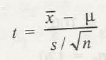t=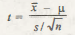1. α = O.OI,

H1 indicates that it is left tailed test. Critical value is 6- 1 which is 5 degrees of the freedom is -3.365

1. Computation

tcal == –2.18

1.  Decision

Since  tcal> -3.365

• It is located in the acceptance region
• H0 is accepted
• The Mean breaking strength of the metallic rope is considered as I60 pounds.

1. b) Testing of Difference of Two Means. In this n1 and n2 are small (< 30) and the population variances are not known but equal and two populations are followed by normal distribution.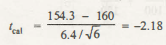1. Setting up H0  : µ1- µ2  = k
2.   Setting up H1  : µ1- µ2 > k,     or µ1- µ2 <  k  or  µ1- µ2 ≠ k
3.   Test statistic

t =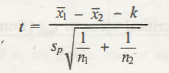Here, the statistic is followed by t – distribution with n1 + n2- 2 degrees of freedom.

1.  Setting up of level of significance, a. and the critical value say t13b at n1 + n2 – 2 degrees of freedom.
2.  Computation of test statistic as tcal·
3. Decision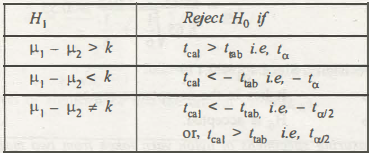H1 Reject H0, if µ1 – µ2 < k tcal>ttab i.e., tα µ1 – µ2> k tcal< -ttab i.e., -tα µ1 – µ2 ≠ k tcal<-ttab i.e., -tα/ 2Or, tcal>ttab i.e., tα/ 2

Example 6. The following are the number of sales with a sample of 6 sales people of gas lighter in a city A and a sample of 8 sales people of gas lighter in another city B made over a certain fixed period of time :

City A :     63,   48,   54,      44,    59,   52

City B :    41,   52,    3850,   66,   54,   44,   61

Assuming that the populations sample is closely related with normal distributions which is having the same variance, test H0 : µ1 = µ2 against H1 :  µ1 ≠ µ2 at the 5% level of significance.

Solution

1. H0 : µ1 = µ2
2. H1 :µ1 ≠ µ2
3. Test statistic:### Customer Reviews

My Homework Help
Rated 5.0 out of 5 based on 510 customer reviews at